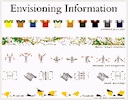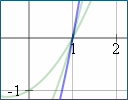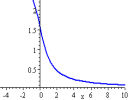Archive for May, 2011

Friday math movie: Artfully visualizing our humanityThis video is a great treatment of math for multiple intelligences - visual, musical and interpersonal.

Visual design for math teachersDiagrams should make things easier to understand, but often they don't. How can we fix this?

Friday math movie: World Metrology Day 2011This video commemorates World Metrology Day, 20th May. Go metric and make your lives simpler!

1. Math tip: Finding a quadratic function from its graph
2. IntMath Poll: What stresses you?
3. Resource: Flat World Knowledge
4. Math tip: Understanding math formulas
5. Is there a place for invention in math?
6. Friday math movies
7. Final thought: perseverance

How to find the equation of a quadratic function from its graphA reader asked how to find the equation of a parabola from its graph.

Friday math movie: Stephen Wolfram on Computing a theory of everythingThe developer of Wolfram|Alpha shares his views on improvements to the normal search engine.

Is there a place for invention in math?Little kids invent things all the time. Should we let them (and their older siblings) invent math?

Friday math movie: Two DotsNow here's an interesting way to think of Euclidean geometry (points, lines and triangles).

IntMath Newsletter: Graph dispute, math resources and capabilities

1. Resource: Top 10 math help sites
2. Which is the correct graph of arccot x?
3. Math tip: Learning math formulas
4. Friday math movie: OK Go This Too Shall Pass
5. Math resource: NSDL
6. Final thought: capabilities

Which is the correct graph of arccot x?Math text books and math software disagree on the correct graph of arccot(x). Which one is right?

Full archive

See the blog sitemap for all previous articles.